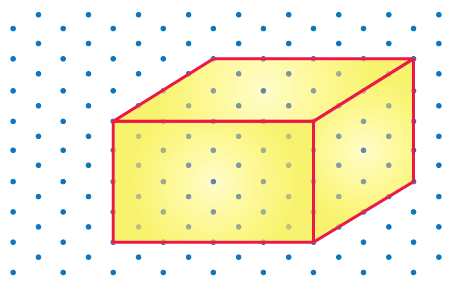## Access Answers to NCERT Class 7 Maths Chapter 15 – Visualising Solid Shapes Exercise 15.2

### Access Answers to NCERT Class 7 Maths Chapter 15 – Visualising Solid Shapes Exercise 15.2

1. Use isometric dot paper and make an isometric sketch for each one of the given shapes:

(i)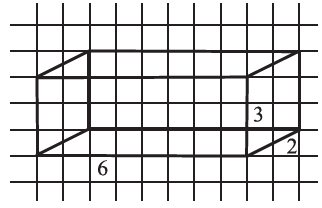Solution:-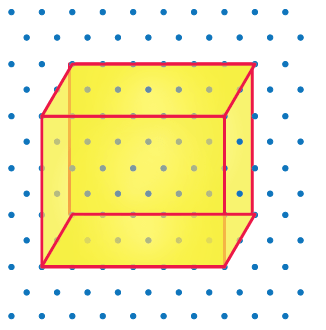(ii)Solution:-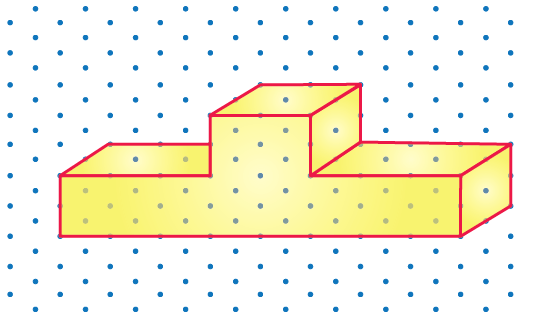(iii)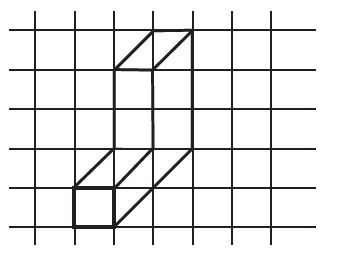Solution:-(iv)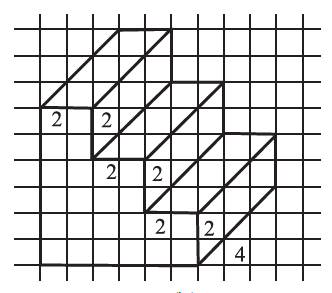Solution:-2. The dimensions of a cuboid are 5 cm, 3 cm and 2 cm. Draw three different isometric sketches of this cuboid.

Solution:-

From the question it is given that dimension of a cuboid are 5 cm, 3 cm, and 2 cm.

The three different isometric sketches of this cuboid is shown below,

12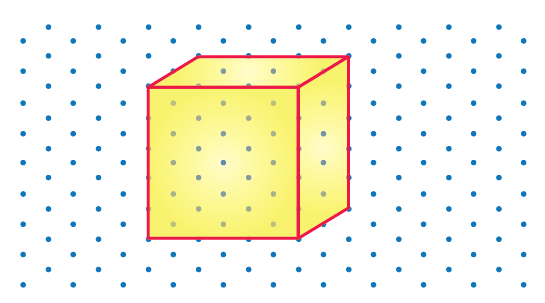3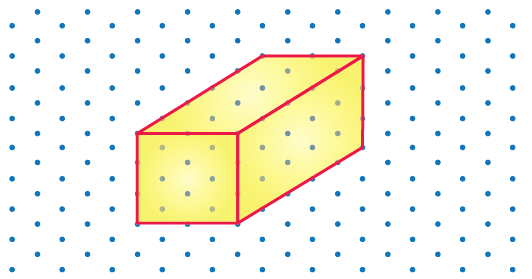3. Three cubes each with 2 cm edge are placed side by side to form a cuboid. Sketch an oblique or isometric sketch of this cuboid.

Solution:-

Oblique sketch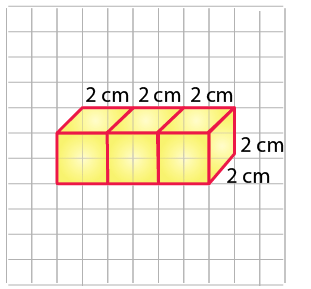Isometric sketch4. Make an oblique sketch for each one of the given isometric shapes:

(i)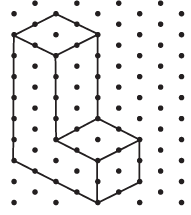Solution:-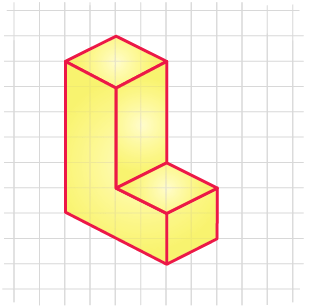(ii)Solution:-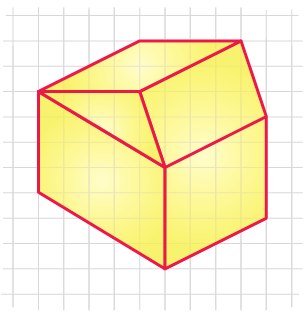5. Give (i) an oblique sketch and (ii) an isometric sketch for each of the following:

(a) A cuboid of dimensions 5 cm, 3 cm and 2 cm. (Is your sketch unique?)

(b) A cube with an edge 4 cm long.

Solution:-

(a)

(i) Oblique sketch(ii) Isometric sketch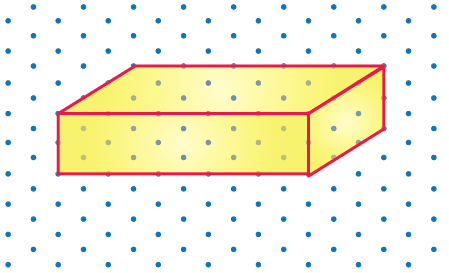(b)

(i) Oblique sketch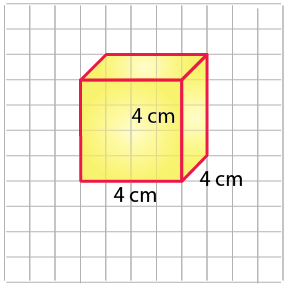(ii) Isometric sketch